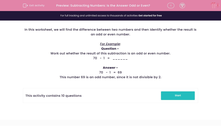# Subtracting Numbers: Is the Answer Odd or Even?

In this worksheet, students subtract one number from another and identify if the answer is an odd or even number.This content is premium and exclusive to EdPlace subscribers.Key stage:  KS 2

Curriculum topic:   Maths and Numerical Reasoning

Curriculum subtopic:   Mixed Problems

Difficulty level:#### Worksheet Overview

In this worksheet, we will find the difference between two numbers and then identify whether the result is an odd or even number.

For Example:

Question -
Work out whether the result of this subtraction is an odd or even number.

70   -  1   =   ______

70   -  1   =  69

This number 69 is an odd number, since it is not divisible by 2.

### What is EdPlace?

We're your National Curriculum aligned online education content provider helping each child succeed in English, maths and science from year 1 to GCSE. With an EdPlace account you’ll be able to track and measure progress, helping each child achieve their best. We build confidence and attainment by personalising each child’s learning at a level that suits them.

Get started••••